Next: Solution: Position of the Up: 3.7 - Simple Potential Previous: Solution: 3D dipole at

## Stream and source: Rankine half-body

It is the superposition of a uniform stream of constant speed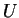and a source of strength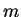.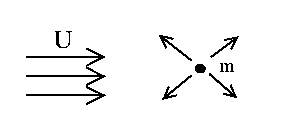2D rankine half-body: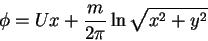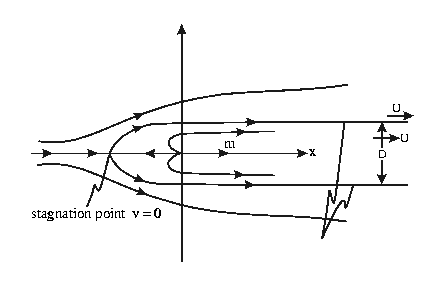3D Rankine half-body: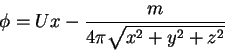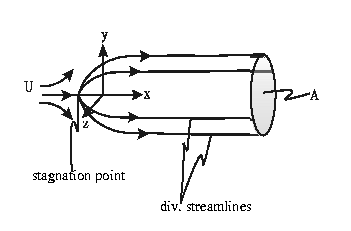1.
Question: find the position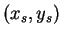of the flow field stagnation point for the 2D Rankine half-body.

(a)
Hint: at the stagnation point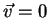.
(b)
Hint: obtain the expression for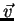and set it equal to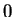, and solve for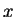and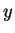.

2.
Question: evaluate the width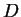of the 2D rankine half-body.

(a)
Hint: mass conservation

3.
Question: find the position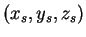of the flow field stagnation point for the 3D Rankine half-body.

(a)
Hint: at the stagnation point.
(b)
Hint: obtain the expression forand set it equal to, and solve for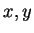and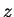.

4.
Question: evaluate the cross-sectional area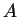of the 3D rankine half-body.

(a)
Hint: mass conservationNext: Solution: Position of the Up: 3.7 - Simple Potential Previous: Solution: 3D dipole at